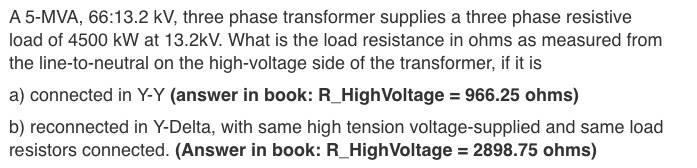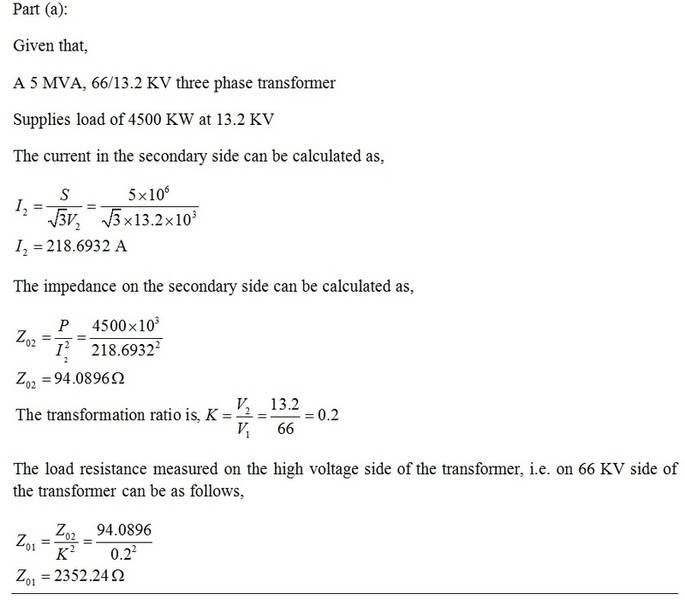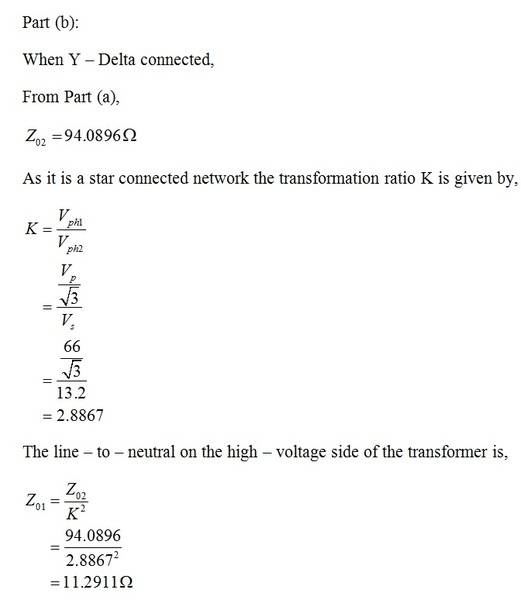# Question with 3 Phase Transformers Wye-Delta problem

## Homework Statement## Homework Equations

Wye:
V_Line = sqrt(3) x V_Phase

Delta:
V_Line = V_Phase

## The Attempt at a SolutionMy answer doesn't quite match up with the solutions in the book, and was wondering what I went wrong here? Any help is greatly appreciated!

## Answers and Replies

Tom.G
Science Advisor
For part a) I get approx. the book answer if the secondary load is 3 phase 4 wire, same configuration as the primary.
You might find it more straightforward to work directly with resistances, rather than with current then calculating resistances.

As the textbooks like to say: "Part b) is left as an exercise for the reader."

For part a) I get approx. the book answer if the secondary load is 3 phase 4 wire, same configuration as the primary.
You might find it more straightforward to work directly with resistances, rather than with current then calculating resistances.

As the textbooks like to say: "Part b) is left as an exercise for the reader."
Yes I've solved it, turned out part b's solution of the book is incorrect according to my professor. Thanks!